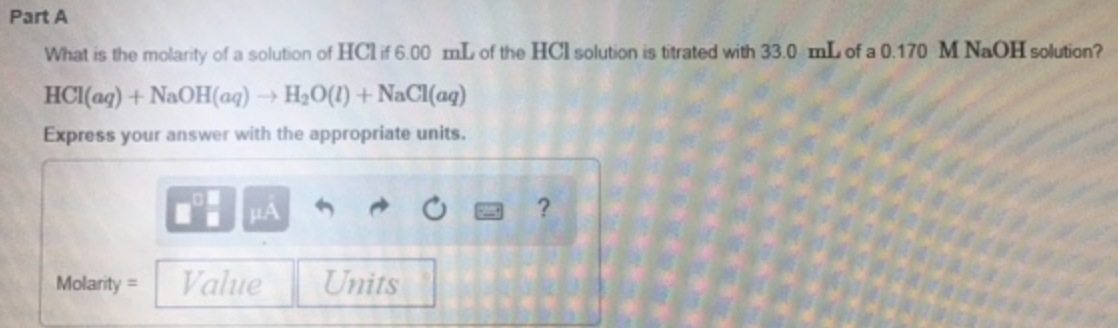# Problem: Part AWhat is the molarity of a solution of HCl if 6.00 mL of the HCl solution is titrated with 33.0 mL of a 0.170 M NaOH solution? HCl (aq) + NaOH (aq) → H2O (l) + NaCl (aq) Express your answer with the appropriate units.

###### FREE Expert Solution
96% (47 ratings)###### Problem Details

Part A

What is the molarity of a solution of HCl if 6.00 mL of the HCl solution is titrated with 33.0 mL of a 0.170 M NaOH solution?

HCl (aq) + NaOH (aq) → H2O (l) + NaCl (aq)